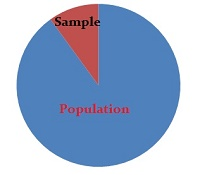# Large Enough Sample Condition

Share on

Statistics Definitions > Large Enough Sample ConditionThe Large Enough Sample Condition tests whether you have a large enough sample size compared to the population. A general rule of thumb for the Large Enough Sample Condition is that n≥30, where n is your sample size. However, it depends on what you are trying to accomplish and what you know about the distribution. In general, the Large Enough Sample Condition applies if any of these conditions are true:

### Central Limit Theorem and the Large Enough Sample Rule

The central limit theorem states that if sample size are large enough, the distribution will be approximately normal. The general rule of n≥30 applies.

### Chi Square and the Large Enough Sample Condition

There are three different tests that use the chi-square ; in each test, the assumptions and conditions are the same, including the Large Enough Sample Condition. To know if your sample is large enough to use chi-square, you must check the Expected Counts Condition: if the counts in every cell is 5 or more, the cells meet the Expected Counts Condition and your sample is large enough. Note that 5 is arbitrary and is open to interpretation. Some texts suggest that it’s okay to have a few expected counts less than 5 (no more than 20%) as long as none are less than 1 (i.e. Yates, Moore & McCabe, The Practice of Statistics, 1999).

### Calculating sample size

Calculating sample size can be one of the most confusing aspects of statistics, mostly because of all the rules (and rules of thumb) surrounding appropriate sizes for different distributions. You have to make sure your sample is sufficiently large, but not too large. For more techniques, see: Sample Size (includes techniques like using tables and calculators).

Reference: Chi-Square John’s Hopkins.

CITE THIS AS:
Stephanie Glen. "Large Enough Sample Condition" From StatisticsHowTo.com: Elementary Statistics for the rest of us! https://www.statisticshowto.com/large-enough-sample-condition/
---------------------------------------------------------------------------Need help with a homework or test question? With Chegg Study, you can get step-by-step solutions to your questions from an expert in the field. Your first 30 minutes with a Chegg tutor is free!